Search IntMath
Close

# Wallis, pi and quantum theory

By Murray Bourne, 24 Nov 2015

In 1656 the English mathematician John Wallis published Arithmetica Infinitorum, which included important ideas that contributed to the development of calculus and an interesting way to find the value of π, called the Wallis Product.

This month, mathematician Tamar Friedmann and physicist Carl Hagen released a surprising new proof of the Wallis Product, arising from an analysis of the energy states of the hydrogen atom.

This Forbes article goes into some detail about the quantum mechanics involved in this new proof: How Pi Connects To Quantum Mechanics.

## Some background on Wallis Product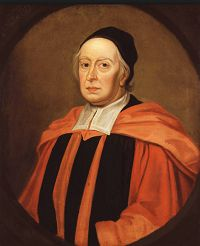John Wallis, 17th century English mathematician [Image source]

Wallis was around 30 years older than Isaac Newton, and his contributions to Newton's later work on calculus were substantial.

Here's an excerpt from one of Wallis' most important works, and one most relevant to this month's new proof of his work: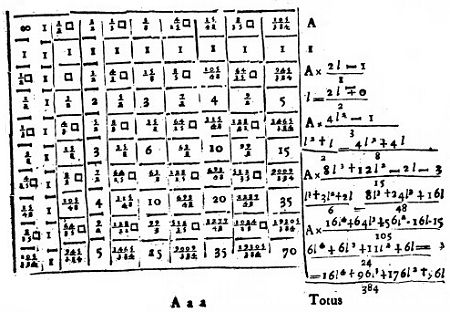A page from Arithmetica Infinitorum. The square symbol stands for 4/π, the ratio of the area of a square to its circumscribed circle. [Image source]

One of the holy grails of 17th century mathematics was to find a solution to the "quadrature of a circle" problem (an attempt to construct a square with the same area as a given circle, using straight edge and compasses only.)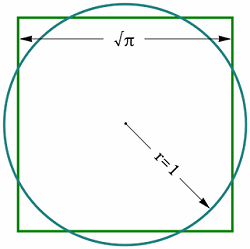Quadrature of the circle problem. How to construct a square of area π? [Image source]

In a section on finding the area of a semicircle by integration, Wallis states an interesting result involving the product of fractions.

Let's look at that result (now called the Wallis Product) one step at a time. It starts with the following product of fractions: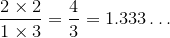Next we take that result and multiply by another fraction in the same pattern, 16/15: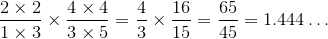Another step, continuing in the same pattern: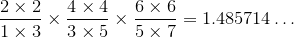We've multiplied by 36/35.

The 4th step is as follows, where we multiply at the end by 64/63: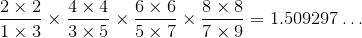The fraction we multiply by gets closer to 1 for each step, and if we keep going, we'll find the value of our fraction products doesn't get any higher than:

1.570796...

In general for this product, we would write (using product notation):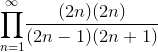Here's a graph of the sequence for n = 1 to n = 20: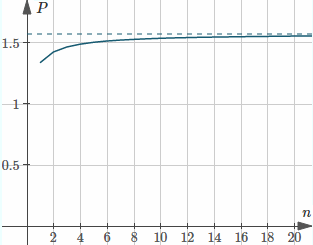Graph of the Wallis Product values for 1 ≤ n ≤ 20

We can see it does slowly approach 1.570796...

## Approximation for π/2

The significance of all this is that 1.570796 is half of π: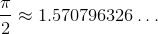So Wallis' Product can give us a good approximation for π.

Here's the way he wrote it in his original publication (it's in Latin, of course, since that was the lingua franca for scientists and mathematicians of the time):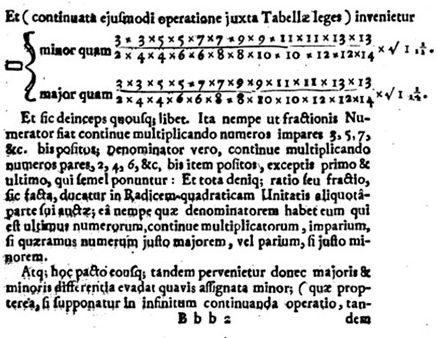Another page from Arithmetica Infinitorum. The fractions at the top are involved in the Wallis Product. [Image source]

### Quantum mechanics proof

The new proof arose out of a consideration of the energy state of the electron in a hydrogen atom.

The new calculation by Friedmann and Hagen involves comparing a known quantity for the lowest energy state by an estimate of the upper bound of the energy state, involving the Gamma Function (which I wrote about here: Factorials and the Gamma Function).

Part of the Wallis Product appears within the Gamma Function expression, and when comparing that to the known state expression, the Wallis Product value of π/2 simply "pops out".

You can see the full details in the article I mentioned before: How Pi Connects To Quantum Mechanics.

### Other achievements by John Wallis

Negative powers: Apart from his contributions to the development of calculus, Wallis was also responsible for the handy notation we use today involving negative indexes giving us powers of fractions, as follows: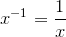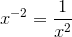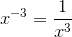Fractional powers: He also gave us the following notation for fractional powers giving us roots: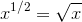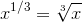(There is some controversy regarding that last equality. See Is cube root the same as raising to power 1/3?.)

Infinity: In an earlier work, Wallis gave us ∞, the symbol we use for infinity.

Analytic treatment of conic sections: The infinity symbol was introduced in one of his other key contributions, the idea that conic sections could be defined analytically. That is, when we cut a cone by a plane at various angles, we get conic sections. (See this Conic Sections Summary.) The formulas we know today for those conic sections are due to Wallis' insight: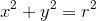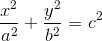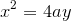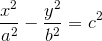Each of these have been described using x- and y-variables, up to power 2.

### Conclusion

John Wallis contributed to many areas of mathematics. It's neat that close to his 400th birthday (he was born on 23 November 1616), a new proof of his product-based approximation for π has been published, and that the proof arises from the study of quantum mechanics of the hydrogen atom.

Hat tip to reader Pi Po for bringing this new proof to my attention.

See the 1 Comment below.

### Comment Preview

HTML: You can use simple tags like <b>, <a href="...">, etc.

To enter math, you can can either:

1. Use simple calculator-like input in the following format (surround your math in backticks, or qq on tablet or phone):
a^2 = sqrt(b^2 + c^2)
(See more on ASCIIMath syntax); or
2. Use simple LaTeX in the following format. Surround your math with $$ and $$.
$$\int g dx = \sqrt{\frac{a}{b}}$$
(This is standard simple LaTeX.)

NOTE: You can mix both types of math entry in your comment.